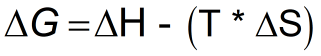Request a Tool

Gibbs Energy Calculator

Combines enthalpy and entropy into a single value

Output

Gibbs free energy (ΔG)
0

Formula• ΔG = is the change in Gibbs free energy
• ΔH = is the change in enthalpy
• ΔS = is the change in entropy
• T = is the temperature in Kelvin

Defination / Uses

Gibbs free energy, denoted G , combines enthalpy and entropy into a single value. The change in free energy, ΔG , is equal to the sum of the enthalpy plus the product of the temperature and entropy of the system. ΔG can predict the direction of the chemical reaction under two conditions:

• Constant Temperature
• Constant Pressure

If ΔG is positive, then the reaction is nonspontaneous (an the input of external energy is necessary for the reaction to occur) and if it is negative, then it is spontaneous (occurs without external energy input).